# 474-Ch7-TransmissionMedia.pptx

May. 29, 2023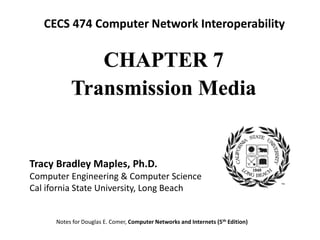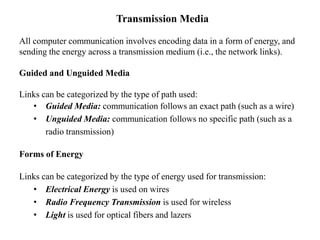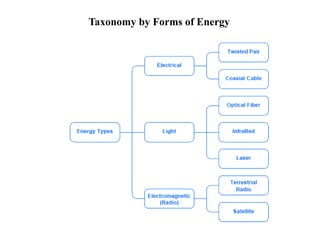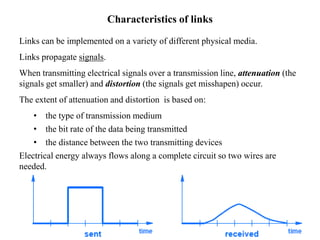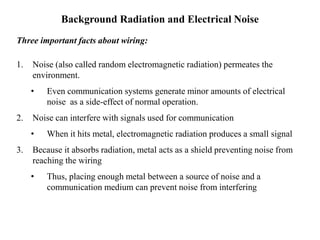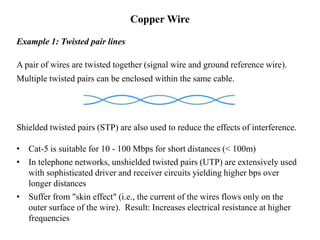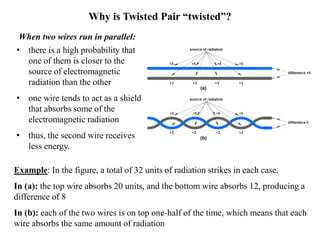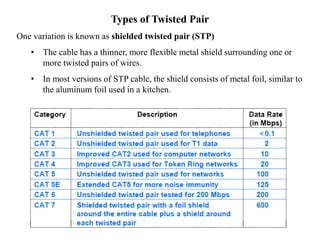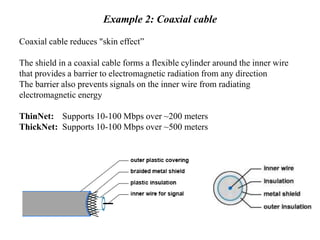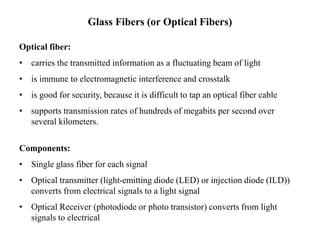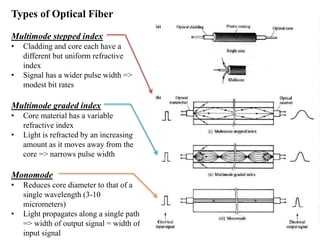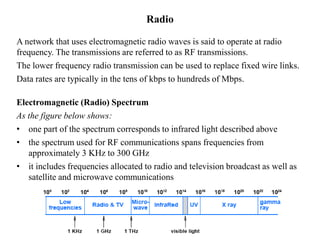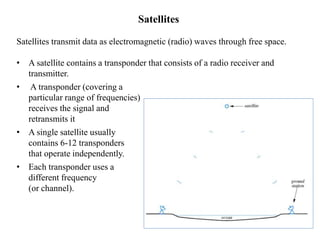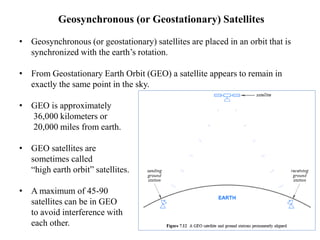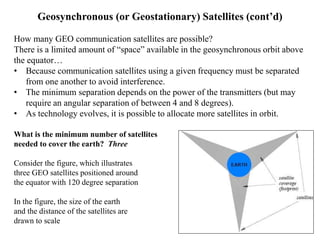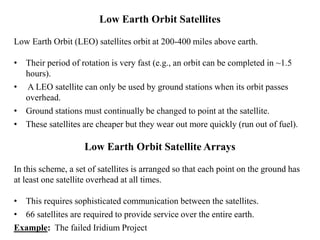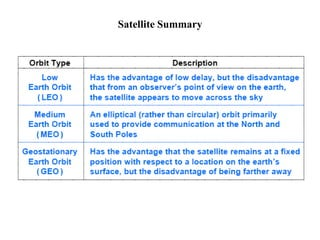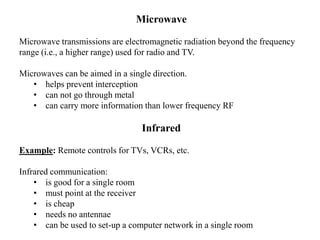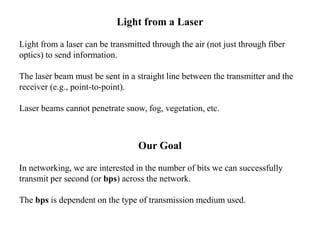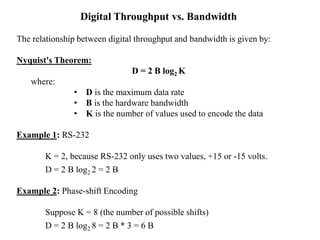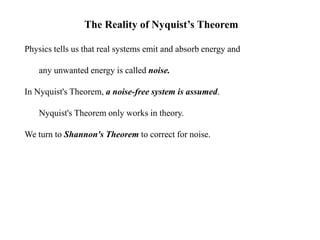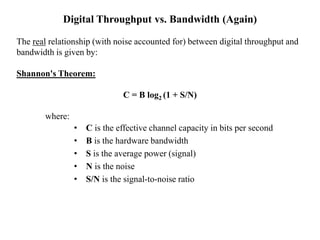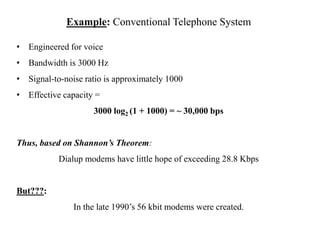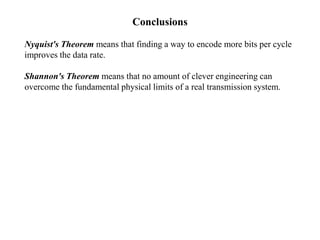1 of 25

### 474-Ch7-TransmissionMedia.pptx

• 1. CHAPTER 7 Transmission Media CECS 474 Computer Network Interoperability Notes for Douglas E. Comer, Computer Networks and Internets (5th Edition) Tracy Bradley Maples, Ph.D. Computer Engineering & Computer Science Cal ifornia State University, Long Beach
• 2. Transmission Media All computer communication involves encoding data in a form of energy, and sending the energy across a transmission medium (i.e., the network links). Guided and Unguided Media Links can be categorized by the type of path used: • Guided Media: communication follows an exact path (such as a wire) • Unguided Media: communication follows no specific path (such as a radio transmission) Forms of Energy Links can be categorized by the type of energy used for transmission: • Electrical Energy is used on wires • Radio Frequency Transmission is used for wireless • Light is used for optical fibers and lazers
• 3. Taxonomy by Forms of Energy
• 4. Characteristics of links Links can be implemented on a variety of different physical media. Links propagate signals. When transmitting electrical signals over a transmission line, attenuation (the signals get smaller) and distortion (the signals get misshapen) occur. The extent of attenuation and distortion is based on: • the type of transmission medium • the bit rate of the data being transmitted • the distance between the two transmitting devices Electrical energy always flows along a complete circuit so two wires are needed.
• 5. Background Radiation and Electrical Noise Three important facts about wiring: 1. Noise (also called random electromagnetic radiation) permeates the environment. • Even communication systems generate minor amounts of electrical noise as a side-effect of normal operation. 2. Noise can interfere with signals used for communication • When it hits metal, electromagnetic radiation produces a small signal 3. Because it absorbs radiation, metal acts as a shield preventing noise from reaching the wiring • Thus, placing enough metal between a source of noise and a communication medium can prevent noise from interfering
• 6. Copper Wire Example 1: Twisted pair lines A pair of wires are twisted together (signal wire and ground reference wire). Multiple twisted pairs can be enclosed within the same cable. Shielded twisted pairs (STP) are also used to reduce the effects of interference. • Cat-5 is suitable for 10 - 100 Mbps for short distances (< 100m) • In telephone networks, unshielded twisted pairs (UTP) are extensively used with sophisticated driver and receiver circuits yielding higher bps over longer distances • Suffer from "skin effect" (i.e., the current of the wires flows only on the outer surface of the wire). Result: Increases electrical resistance at higher frequencies
• 7. When two wires run in parallel: • there is a high probability that one of them is closer to the source of electromagnetic radiation than the other • one wire tends to act as a shield that absorbs some of the electromagnetic radiation • thus, the second wire receives less energy. Example: In the figure, a total of 32 units of radiation strikes in each case. In (a): the top wire absorbs 20 units, and the bottom wire absorbs 12, producing a difference of 8 In (b): each of the two wires is on top one-half of the time, which means that each wire absorbs the same amount of radiation Why is Twisted Pair “twisted”?
• 8. Types of Twisted Pair One variation is known as shielded twisted pair (STP) • The cable has a thinner, more flexible metal shield surrounding one or more twisted pairs of wires. • In most versions of STP cable, the shield consists of metal foil, similar to the aluminum foil used in a kitchen.
• 9. Example 2: Coaxial cable Coaxial cable reduces "skin effect” The shield in a coaxial cable forms a flexible cylinder around the inner wire that provides a barrier to electromagnetic radiation from any direction The barrier also prevents signals on the inner wire from radiating electromagnetic energy ThinNet: Supports 10-100 Mbps over ~200 meters ThickNet: Supports 10-100 Mbps over ~500 meters
• 10. Glass Fibers (or Optical Fibers) Optical fiber: • carries the transmitted information as a fluctuating beam of light • is immune to electromagnetic interference and crosstalk • is good for security, because it is difficult to tap an optical fiber cable • supports transmission rates of hundreds of megabits per second over several kilometers. Components: • Single glass fiber for each signal • Optical transmitter (light-emitting diode (LED) or injection diode (ILD)) converts from electrical signals to a light signal • Optical Receiver (photodiode or photo transistor) converts from light signals to electrical
• 11. Types of Optical Fiber Multimode stepped index • Cladding and core each have a different but uniform refractive index • Signal has a wider pulse width => modest bit rates Multimode graded index • Core material has a variable refractive index • Light is refracted by an increasing amount as it moves away from the core => narrows pulse width Monomode • Reduces core diameter to that of a single wavelength (3-10 micrometers) • Light propagates along a single path => width of output signal = width of input signal
• 13. Radio A network that uses electromagnetic radio waves is said to operate at radio frequency. The transmissions are referred to as RF transmissions. The lower frequency radio transmission can be used to replace fixed wire links. Data rates are typically in the tens of kbps to hundreds of Mbps. Electromagnetic (Radio) Spectrum As the figure below shows: • one part of the spectrum corresponds to infrared light described above • the spectrum used for RF communications spans frequencies from approximately 3 KHz to 300 GHz • it includes frequencies allocated to radio and television broadcast as well as satellite and microwave communications
• 14. Satellites Satellites transmit data as electromagnetic (radio) waves through free space. • A satellite contains a transponder that consists of a radio receiver and transmitter. • A transponder (covering a particular range of frequencies) receives the signal and retransmits it • A single satellite usually contains 6-12 transponders that operate independently. • Each transponder uses a different frequency (or channel).
• 15. Geosynchronous (or Geostationary) Satellites • Geosynchronous (or geostationary) satellites are placed in an orbit that is synchronized with the earth’s rotation. • From Geostationary Earth Orbit (GEO) a satellite appears to remain in exactly the same point in the sky. • GEO is approximately 36,000 kilometers or 20,000 miles from earth. • GEO satellites are sometimes called “high earth orbit” satellites. • A maximum of 45-90 satellites can be in GEO to avoid interference with each other.
• 16. Geosynchronous (or Geostationary) Satellites (cont’d) How many GEO communication satellites are possible? There is a limited amount of “space” available in the geosynchronous orbit above the equator… • Because communication satellites using a given frequency must be separated from one another to avoid interference. • The minimum separation depends on the power of the transmitters (but may require an angular separation of between 4 and 8 degrees). • As technology evolves, it is possible to allocate more satellites in orbit. What is the minimum number of satellites needed to cover the earth? Three Consider the figure, which illustrates three GEO satellites positioned around the equator with 120 degree separation In the figure, the size of the earth and the distance of the satellites are drawn to scale
• 17. Low Earth Orbit Satellites Low Earth Orbit (LEO) satellites orbit at 200-400 miles above earth. • Their period of rotation is very fast (e.g., an orbit can be completed in ~1.5 hours). • A LEO satellite can only be used by ground stations when its orbit passes overhead. • Ground stations must continually be changed to point at the satellite. • These satellites are cheaper but they wear out more quickly (run out of fuel). Low Earth Orbit Satellite Arrays In this scheme, a set of satellites is arranged so that each point on the ground has at least one satellite overhead at all times. • This requires sophisticated communication between the satellites. • 66 satellites are required to provide service over the entire earth. Example: The failed Iridium Project
• 19. Microwave Microwave transmissions are electromagnetic radiation beyond the frequency range (i.e., a higher range) used for radio and TV. Microwaves can be aimed in a single direction. • helps prevent interception • can not go through metal • can carry more information than lower frequency RF Infrared Example: Remote controls for TVs, VCRs, etc. Infrared communication: • is good for a single room • must point at the receiver • is cheap • needs no antennae • can be used to set-up a computer network in a single room
• 20. Light from a Laser Light from a laser can be transmitted through the air (not just through fiber optics) to send information. The laser beam must be sent in a straight line between the transmitter and the receiver (e.g., point-to-point). Laser beams cannot penetrate snow, fog, vegetation, etc. Our Goal In networking, we are interested in the number of bits we can successfully transmit per second (or bps) across the network. The bps is dependent on the type of transmission medium used.
• 21. Digital Throughput vs. Bandwidth The relationship between digital throughput and bandwidth is given by: Nyquist's Theorem: D = 2 B log2 K where: • D is the maximum data rate • B is the hardware bandwidth • K is the number of values used to encode the data Example 1: RS-232 K = 2, because RS-232 only uses two values, +15 or -15 volts. D = 2 B log2 2 = 2 B Example 2: Phase-shift Encoding Suppose K = 8 (the number of possible shifts) D = 2 B log2 8 = 2 B * 3 = 6 B
• 22. The Reality of Nyquist’s Theorem Physics tells us that real systems emit and absorb energy and any unwanted energy is called noise. In Nyquist's Theorem, a noise-free system is assumed. Nyquist's Theorem only works in theory. We turn to Shannon's Theorem to correct for noise.
• 23. Digital Throughput vs. Bandwidth (Again) The real relationship (with noise accounted for) between digital throughput and bandwidth is given by: Shannon's Theorem: C = B log2 (1 + S/N) where: • C is the effective channel capacity in bits per second • B is the hardware bandwidth • S is the average power (signal) • N is the noise • S/N is the signal-to-noise ratio
• 24. Example: Conventional Telephone System • Engineered for voice • Bandwidth is 3000 Hz • Signal-to-noise ratio is approximately 1000 • Effective capacity = 3000 log2 (1 + 1000) = ~ 30,000 bps Thus, based on Shannon’s Theorem: Dialup modems have little hope of exceeding 28.8 Kbps But???: In the late 1990’s 56 kbit modems were created.
• 25. Conclusions Nyquist's Theorem means that finding a way to encode more bits per cycle improves the data rate. Shannon's Theorem means that no amount of clever engineering can overcome the fundamental physical limits of a real transmission system.